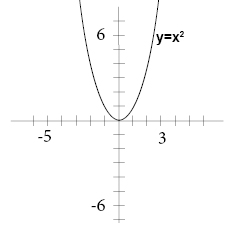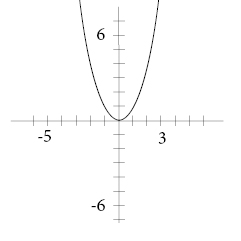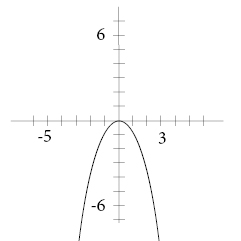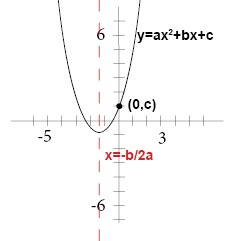# How do you graph F(x)=3x^2+4x-1 ?

Apr 28, 2018

Find the vertex and plot some "nice" points around that point.

#### Explanation:

$y = 3 {x}^{2} + 4 x - 1$
$a = 3 , b = 4 , \mathmr{and} c = - 1$
The vertex is at ${x}_{v} = - \frac{b}{2 a} = - \frac{4}{6} = - \frac{2}{3}$
The corresponding y value is at
${y}_{v} = 3 {\left(- \frac{2}{3}\right)}^{2} + 4 \left(- \frac{2}{3}\right) - 1 = \frac{4}{3} - \frac{8}{3} - \frac{3}{3} = - \frac{7}{3}$

Find some "nice" values of x that are around the vertex.

$f \left(- 2\right) = 12 - 8 - 1 = 3$
$f \left(- 1\right) = 3 - 4 - 1 = - 2$
$f \left(0\right) = 0 + 0 - 1 = - 1$
$f \left(1\right) = 3 + 4 - 1 = 6$

Plot the points $\left(- 2 , 3\right) , \left(- 1 , - 2\right) , \left(- \frac{2}{3} , - \frac{7}{3}\right) , \left(0 , - 1\right) , \mathmr{and} \left(1 , 6\right)$ and sketch in the graph. graph{3x^2+4x-1 [-3, 1.2, -4, 7]}

Apr 29, 2018

#### Explanation:

show the steps

For example $a {x}^{2} + b x + c = 0$

A nonlinear function that can be written on the standard form

$a {x}^{2} + b x + c , \setminus : \setminus : w h e r e \setminus : \setminus : a \setminus \ne q 0$

All quadratic functions has a U-shaped graph called a parabola. The parent quadratic function is

$y = {x}^{2}$The lowest or the highest point on a parabola is called the vertex. The vertex has the x-coordinate

$x = - \setminus \frac{b}{2 a}$

The y-coordinate of the vertex is the maximum or minimum value of the function.

$a > 0$ parabola opens up$a < 0$ parabola opens downA rule of thumb reminds us that when we have a positive symbol before x2 we get a happy expression on the graph and a negative symbol renders a sad expression.

The vertical line that passes through the vertex and divides the parabola in two is called the axis of symmetry. The axis of symmetry has the equation

$x = - \setminus \frac{b}{2 a}$

The y-intercept of the equation is c.When you want to graph a quadratic function you begin by making a table of values for some values of your function and then plot those values in a coordinate plane and draw a smooth curve through the points.

now we will graph

$F \left(x\right) = 3 {x}^{2} + 4 x - 1$donot forget Make a table of value for some values of x. Use both positive and negative values!